# 针对交易的组合数学和概率论（第一部分）：基础知识

20 九月 2021, 08:03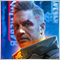0
1 979

### 运用概率论进行手动和自动交易的细节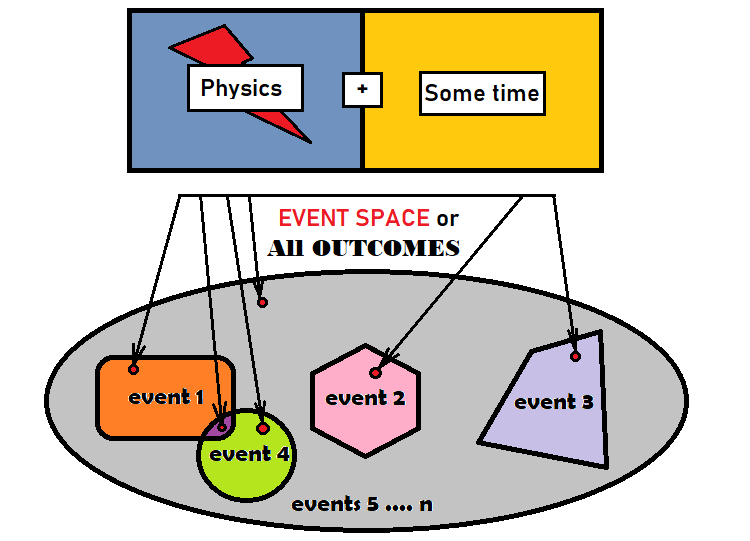1. 可能产物的数量有限
2. 可能产物的数量无限

• P = S/N , S 是满足事件标准的产物数量，N 是事件空间中所有产物的总数

• N = S + S + ... + S[n]
• S 是特定事件的产物数量
• N 是事件空间的所有产物

• 1 = S/N   +   S/N   +   ...   +S[n]/N

• 1  = P   +   P   +   ...   +P[n]

• R = R(X,Y)
• R 是子弹命中坐标点 (X,Y) 的概率密度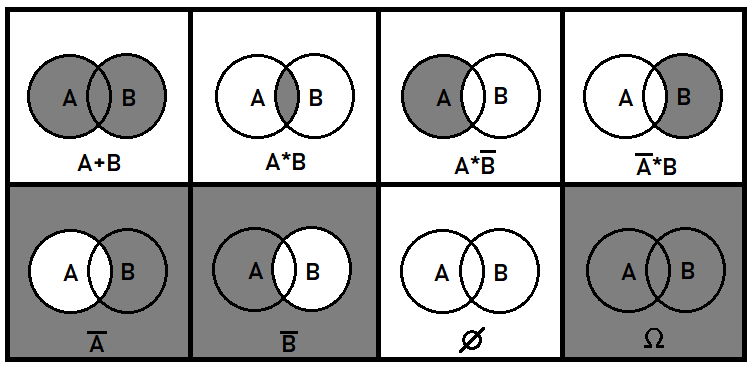### 概率树和假设

• P(A) = Sum(0 ... i .... n) [ P(H[i]) * P(A|H[i]) ] - 事件 A 的概率
• P(H[i]) — 假设概率 H[i]
• P(A|H[i]) — 事件 A 在假设 H[i] 框架内发生的概率

1. 相对于空间 H[i] 计算出的事件 H[k] 的概率

• P(H[i]|O) — 因为这个概率是相对于 O 估算的。

• P(A) = (S + ... + S[i] + ... + S[n]) / O   =   S/O + ... + S[i]/O + ... + S[n]/O  =  (S/N) * ( N/O ) + ... + (S[i]/N[i]) * ( N[i]/O ) + ... + (S[n]/N[n]) * ( N[n]/O )
• S[i] — 假设 H[i] 交集特定片段的面积
• N[i]  — 整个假设 H[i]（包括 S[i]）的面积
• O  — 所有结果或整个椭圆的面积

• S[i]/N[i] ----> P(A|H[i])
• N[i]/O ----> P(H[i])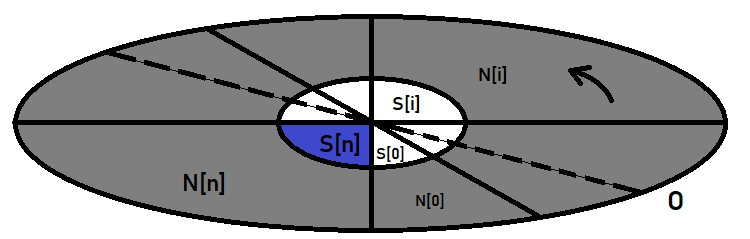• P(A) = P(H) * P(A|H)
• H  — 假设的概率，所选事件完全处于其内。

• P(B) = P(A) * P(B|A) = P(H) *  P(A|H) *  P(B|A)

### 关于分形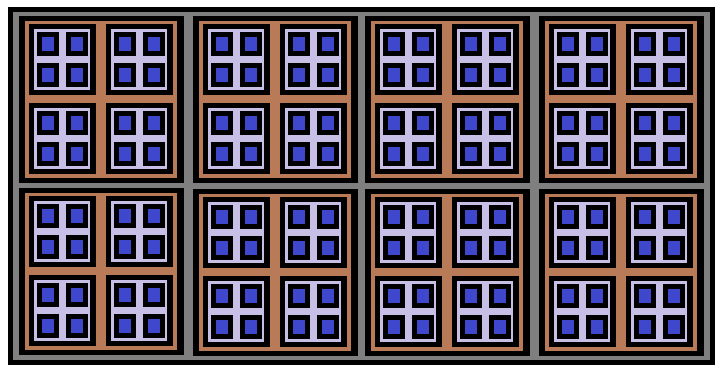• n! - 数值 n 的阶乘
• P(n,k) = n! / ( n - k )!  — 从 N 个元素到 K 个元素的排列
• С(n,k) = n! / ( k! * ( n - k )! )  — N 个元素乘 K 个元素的组合

• P(n,k) = C(n,k) * P(k,k)

• C(n,k) = P(n,k)/P(k,k) = n! / ( k! * ( n - k )! )

### 伯努利（Bernoulli）预划案

• P = C(n,k)*Pow(p,k)*Pow(q,n-k)   — 伯努利公式
• p  — 单次实验结果中事件发生的概率
• q = 1 - p   — 实验结果中事件不会发生的概率

• P(n) = P(H|O) *  P(H|H) *  P(H|H) * ... * P(H[k]|H[k-1]) * ... *P(H[n]|H[n-1])
• n  — 链中的段数
• O  — 整个产物集合；可以表示为 H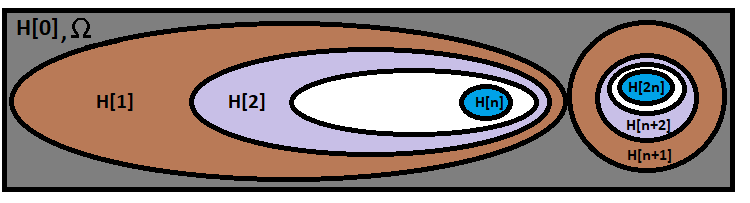• n+1 (因为 “0” 的成功产物组合也要被计算在内)
• n 是实验链中独立测试的数量

• P(n) = Pow(p,k)*Pow(q,n-k)
• k  — 乘积中有多少个等于 “p” 的因数
• n-k  — 乘积中有多少个等于 “q” 的因数

• P = C(n,k)*Pow(p,k)*Pow(q,n-k)

• P = Summ(k+1 ... i ... n)[C(n,i)*Pow(p,i)*Pow(q,n-i)]

• P = Summ(0 ... i ... n)[C(n,i)*Pow(p,i)*Pow(q,n-i) ] = 1

• Summ(0 ... i ... n)[C(n,i)] = Pow(2,n)

• C(n,k) =  C(n,n-k)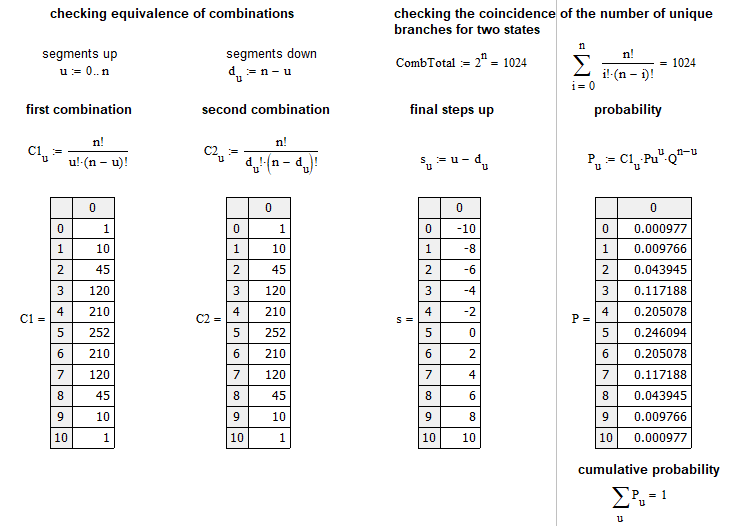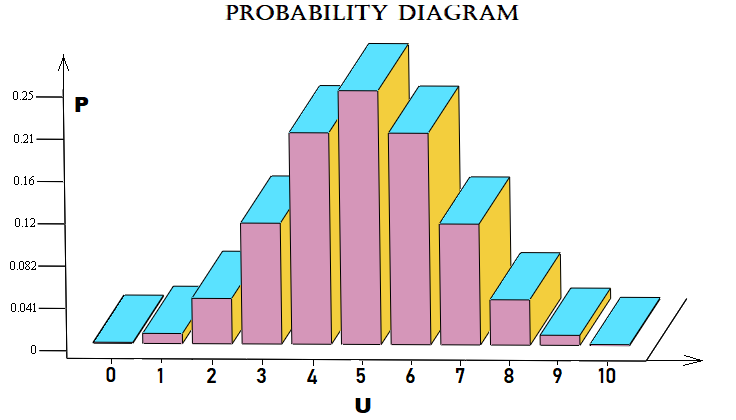### 创建第一个分形

• P(TP) = SL / ( TP + SL ) - 触发止盈的概率
• P(SL) = TP / ( TP + SL ) - 触发止损的概率
• SL  — 止损点距
• TP  — 止盈点距

• P(TP) + P(SL) = 1

• M =  P(TP) * TP - P(SL) * SL = 0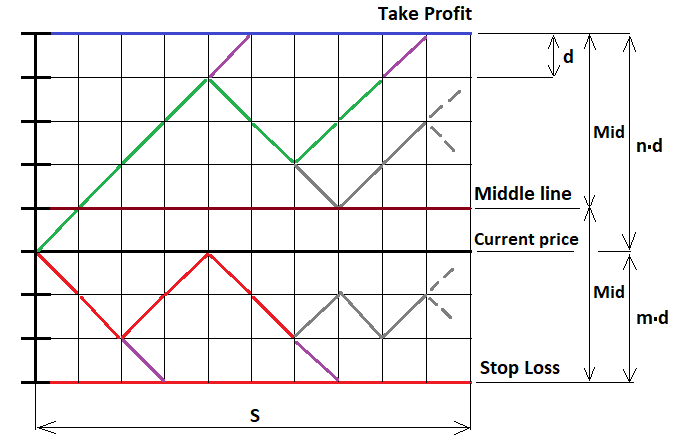1. 我们在中线上方（ U > MiddleLine ）
2. 我们在中线下方 ( U < MiddleLine )
3. 我们处于中线之上 ( U = MiddleLine )

“U” 是相对于开盘价之上 “u-d” 的总步级数。 如果我们要继续构建分形时点距低于价格，则 U 根据函数取负值。 如果我们位于中线，则我们可取的步级数不用担心交叉线低于 Mid。 但是在继续构建之前，我们必须将分形构建限制为价格或订单可以执行的步级数。 如果步级数超过了要求数量，我们必须中断进一步的构建。 否则，我们将得到无限递归，无法退出。 它的计算时间将等于无穷大。

• (n - 1) - U  — 当我们的链已高于中线之上时（因为 U 的增加会导致到上边界的距离减少）
• (m - 1) + U  — 当我们的链已低于中线之下时（因为 U 的减少会导致到下边界的距离减少）
• (floor(Mid)-1)  — 当我们的链条正好处于中线时
• n  — 顶部段落数量
• m  — 底部段落数量
• floor  — 该函数舍弃小数部分（这可能没有必要）

• Mid = (m+n)/2  — 范围宽度的一半（步级数）
• Middle = (m+n)/2 - m   — 中线的 “U” 值（步级数）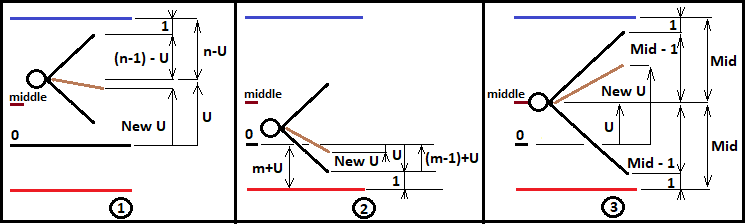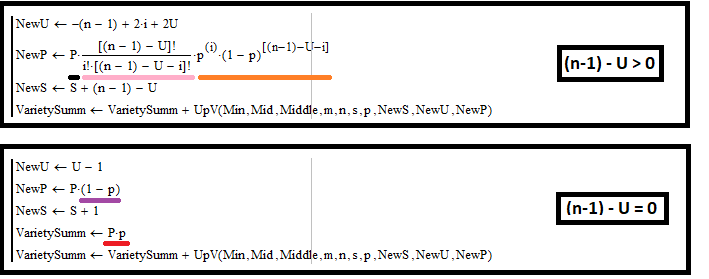• ( n - 1 ) - U = f — 它是未来组合树的步级数（距离由到分形范围最近边界的距离决定）
• u + d = f  — 数值相同，但表达形式为上涨和下跌段落的数量
• s = u - d  — 最终步级数量，以下跌段落和上涨段落表示
• u = i  — 这是因为我的程序使用 “i” 迭代（应该记住）

1. d = f - u = f - i = ( n - 1 ) - U - i
2. s = i -( n - 1 ) + U + i = -( n - 1 ) + 2*i + U

• NewU = s + U = -( n - 1 ) + 2*i + 2*U   - 我们要传递到下一个分形级别的新 “U”
• NewP = P * C(f,i) * Pow(p,i) * Pow(1-p,f-i)P * C( ( n - 1 ) - U ,i) * Pow(p,i) * Pow(1-p,( n - 1 ) - U -i)  — 我们新的链概率 “P” 被传递 到下一个分形级别（通过乘以链的新段落概率获得）
• NewS = S + f = S + ( n - 1 ) - U  — 新的 “S” 要传递到下一个分形级别

1. 收集与边界相交链的概率（红色下划线）
2. 类似的动作，把我们将传递到下一个分形级别的新值提升

1. 触及边界
2. 从边界翻转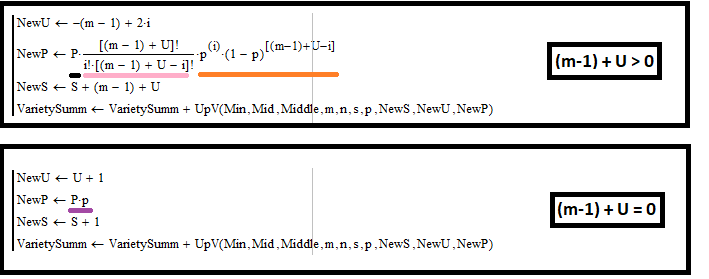• ( m - 1 ) + U = f

1. d = f - u = f - i = ( m - 1 ) + U - i
2. s = i -( m - 1 ) - U + i = -( m - 1 ) + 2*i - U

• NewU = s + U = -( m - 1 ) + 2*i  - 我们的新 “U” 将被传递到下一个分形级别
• NewP = P * C(f,i) * Pow(p,i) * Pow(1-p,f-i) P * C( ( m - 1 ) + U ,i) * Pow(p,i) * Pow(1-p,( m - 1 ) + U -i)  — 我们将链 “P” 的新概率传递到下一个分形层
• NewS = S + f = S + ( m - 1 ) + U  — 我们的新 “S” 将被传递到下一个分形层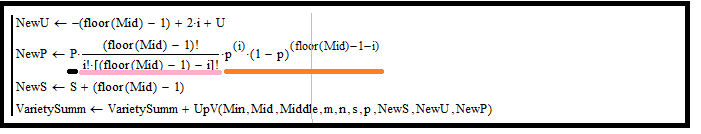• floor(Mid) - 1 = f

1. d = f - i =  floor(Mid) - 1 - i
2. s = i - d = -(floor(Mid) - 1) + 2*i

• NewU = s + U = -(floor(Mid) - 1) + 2*i + U
• NewP = P * C(f,i) * Pow(p,i) * Pow(1-p,f-i) = P * C( floor(Mid) - 1 ,i) * Pow(p,i) * Pow(1-p,floor(Mid) - 1 - i)  — 我们的新链概率“P”，将被传递到下一个分形级别
• NewS = S + f = S + (floor(Mid) - 1) — 我们的新 “S”，将被传递到下一个分形级别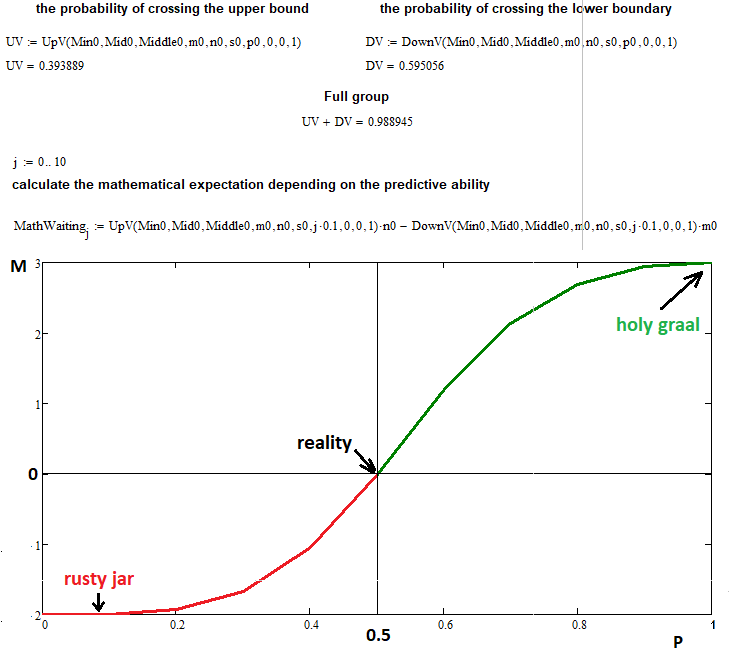• n = 3 — 顶部段落数量
• m = 2  — 底部段落数量
• s = 22  — 分形函数链式反应的允许步级数量（如果您增加这个数值，它会给计算机带来额外的负担，所以这个步级数量已经足够了）
• 0 ... 0.1 ... 0.2 ...... 1.0   — 将上升步级概率的范围切分为 10 个部分，步长为 0.1

### 总结

• 概率论与程序设计相结合，可为描述众多行情过程提供理论基础。
• 分形结合概率论的主要法则可以解答最困难的问题。
• 我们已经见识到了一个创建相当复杂分形的例子。
• 文章已证明伯努利预划案为创建任何具有两步状态的分形提供了机会。

### 结束语

我希望读者能从这份资料中看到一些新的东西，可以在实践中用于交易。 在撰写本文时，我尝试传达离散数学和概率论的全部力量，从而令您为另一项艰巨的任务做好准备 — 运用分形概率链描述市场过程。 我尝试将概率论的所有主要法则组合成一种素材，其可进一步帮助解决实际交易中的复杂任务。 我还尝试删除所有无关紧要的细节。 下一篇文章将提供分形实际应用的新示例，以及其它重要问题的解答。

本文译自 MetaQuotes Software Corp. 撰写的俄文原文
原文地址： https://www.mql5.com/ru/articles/9456

附加的文件 |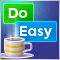DoEasy 函数库中的图形（第七十九部分）：“动画框”对象类及其衍生对象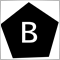优秀程序员（第 01 部分）：您必须停止做这 5 件事才能成为一名成功的 MQL5 程序员DoEasy 函数库中的图形（第八十部分）：“几何动画框”对象类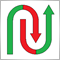形态与示例（第一部分）：多顶